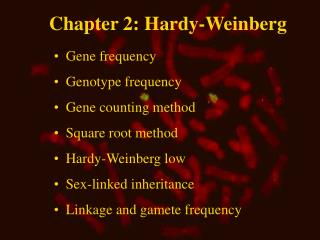DownloadDownload PresentationChapter 2: Hardy-Weinberg

# Chapter 2: Hardy-Weinberg

Download Presentation## Chapter 2: Hardy-Weinberg

- - - - - - - - - - - - - - - - - - - - - - - - - - - E N D - - - - - - - - - - - - - - - - - - - - - - - - - - -
##### Presentation Transcript

1. Chapter 2: Hardy-Weinberg • Gene frequency • Genotype frequency • Gene counting method • Square root method • Hardy-Weinberg low • Sex-linked inheritance • Linkage and gamete frequency

2. Co-dominant inheritance • S is the ”slow” albumin allele • F is the ”fast” albumin allele Genotyper

3. Genotype frequency

4. Calculation of genotype frequencies • Genotype frequency of SS: 36/106 = 0.34 • Genotype frequency of SF: 47/106 = 0.44 • Genotype frequency of FF: 23/106 = 0.22

5. Calculation of gene frequencies • Gene frequency derived from numbers • Gene frequency derived from proportions

6. Gene frequency derived from numbers • S: p = (236+47)/(2106) = 0.56 • F: q = (223+47)/(2106) = 0.44 • Total: p + q = 1.00

7. Gene frequency derived from proportions • S: p = 0.34+0.50.44 = 0.56 • F: q = 0.22+0.50.44 = 0.44 • Total: p + q = 1.00

8. Multiple alleles • The calculation of gene frequency for more than two alleles

9. Gene frequency calculation for multiple alleles • Allele frequency of ”209”: p = (22+18)/(243) = 0.256 • Allele frequency of ”199”: q = (20+12)/(243) = 0.140 • Allele frequency of ”195”: r = 1 - p - q = 0.604

10. Dominant inheritance

11. Gene frequency calculation for dominant inheritance • q 2 = qq = 18/200 = 0.09 • q = qq = 0.30 • p = 1-q = 1-0.30 = 0.70

12. Hardy-Weinberg law • The frequency of homozygotes is equal to the gene frequenciessquared: p2 og q2 • The frequency of heterozygotes is equal to twice the product of the two gene frequencies: 2pq • Gene- and genotype frequencies are constant from one generation to the next

13. Hardy-Weinberg law Genotypefrekvens: • SS: pp = 0.560.56 = 0.314 • FF: qq = 0.440.44 = 0.194 • SF: 2pq = 2  0.560.44 = 0.493

14. 2-test for H-W equilibrium • H0: No difference between observed and expected numbers • 2 = S (O-E)2/E = 1.09 • Significant level: a = 0.05 • Degrees of freedom: df = 1

15. 2-test • P > 0.20 P > a • H0 is not rejected. There is no significant difference between observed and expected numbers Conclusion: There is no significant deviation from Hardy-Weinberg equlibrium for albumintype inDanish German Shepherd dogs

16. Sex-linked inheritanceX-linkage • Males an females do not necessarily contain the same gene frequencies • The mammalian male’s X chromosome comes from the mother • In the mammalian male expression of the gene is direct, i.e. the genotype frequency is equal to the gene frequency • The genotype in the male is called a hemizygote

17. The Orange gene in cats • XX-individuals: OO gives orange coat colour Oo gives mixed coat colour oo gives no orange colour in the coat • XY-individuals: • O gives orange coat colour • o gives no orange colour in the coat

18. Calculation of the frequency of the orange gene in cats • Ofemale: p = (23+53)/(2173) = 0.17 • ofemale: q = (2117+53)/(2173) = 0.83 • Omale: p = 28/177 = 0.16 • omale: q = 149/177 = 0.84

19. Sex-linked inheritance • Sex-linked recessive diseases can be expected to occur at a higher frequency in males compared to the females • Males: Gene frequency q = 0.01 Genotype frequency = Gene frequency • Females: Gene frequency q = 0.01 Genotype frequency = q2 = 0.0001

20. Mating type frequencies at random matingMating type FrequencyAA  AA p2 p2 = p4AA  Aa 2  p2 2pq = 4p3 qAA  aa 2  p2 q2 = 2p2 q2 Aa  Aa 2pq  2pq = 4p2  q2 Aa  aa 2 2pq q2 = 4pq3 aa  aa q2 q2 = q4

21. Mono genetic inherited diseases Closely related dog breeds: gene frequencies Mating type frequencies

22. Gamete frequencies, linkage and linkage disequilibrium • Gamete frequencies are used when two genes at two loci are studied simultaneously • A marker allele always occurs with a harmful gene on the other locus

23. Gamete frequencies by linkage fits into a two by two table Linkage Rekombination Repulsion Genotypeprocess Genotype A B A B A b a b a b a B

24. Gamete frequencies by linkage: Calculation example • Test for independence • H0: D = 0, c2 = 9.7, df = 1, a = 0.05 • H0 rejected  linkage disequilibrium • D = r - p(A)  p(B) = 0.21-0.7  0.4 = - 0.07

25. Gamete frequencies by linkage • The gametes Ab og aB are in repulsions phase • Obs. - Exp. = deviation = D

26. Gamete frequencies by linkage Linkage Rekombination Repulsion Genotypeprocess Genotype A BA b ab aB a b a b a B

27. Linkage disequilibrium • Obs - Exp = deviation = D • D = u - q(a)q(b), or D = ru - ts (= (f (AB/ab) - f (Ab/aB))/2 ) • Maximum disequilibrium (Dmax) occurs when all double heterozygotes are either in linkage phase (AB/ab) or in repulsions phase (Ab/aB). Dmax = 0.5

28. Disappearance of linkage disequilibrium • Dn = D0(1-c)n, where D0 is the linkage disequilibrium in the base population

29. Gamete frequencies at linkage disequilibrium and equilibrium • In connection with a new mutation, linkage disequilibrium occurs in many of the following generations, as the mutation only arises in one chromosome • There is always maximum linkage disequilibrium within a family